# Heat Pump

A heat pump is basically a heat engine run in the reverse direction. In other words, a heat pump is a device that is used to transfer heat energy to a thermal reservoir. They are often used to transfer thermal energy by absorbing heat from a cold space and releasing it to a warmer one.

Heat pumps transfer heat from cold body hot body by the expense of mechanical energy supplied to it by an external agent. The cold body is cooled more and more. A heat pump generally comprises four key components which include a condenser, a compressor, an expansion valve and an evaporator. The working substance used in these components is called refrigerant.

Some of the most common examples of a heat pump include freezers and air conditioners, or other heating, ventilating devices. As for the applications, heat pumps are used either for heating or cooling.

## How to find the Coefficient of Performance of a Heat Pump

When we talk about heat pump efficiencies we basically have to deal with a few key terms such as;

• Coefficient of performance (COP)
• Seasonal coefficient of performance (SCOP)
• Seasonal performance factor (SPF)

The performance of the heat pump is expressed by the coefficient of performance (K).

K = Heat extracted from the cold body / Work need to transfer it to the hot body

K = Heat extracted by work done

K = QL / W

From 1st law of thermodynamics for the cyclic process ΔU = 0

ΔQ = ΔW

W = QH – QL

K = QL / QH – QL

Note: If a heat pump has a higher number then it will be more efficient and it will consume less energy. It is cost-effective to operate. However, factors such as auxiliary equipment, size, control system, technology affect the efficiency of a heat pump.

Additionally, conditions such as temperature and humidity also need to be considered. Efficiency decreases if temperature difference increases or when freezing takes place.

## Related Terms

Thermal reservoir: A thermal reservoir is a huge body having a great heat capacity in such a way that a finite quantity of heat can be added to it (or) removed from it without changing its temperature.

Heat source (or) Hot reservoir: It is a thermal reservoir used in a heat engine for supplying heat at high temperature.

Heat sink: This is a thermal reservoir used in a heat engine for receiving the remaining heat at low temperature.

Heat engine: It is a cyclically operating energy conversion device. A heat engine‘s main objective is converting heat into work. Source supplies an amount of heat Q1 to the working body which converts some of it into work ‘W’ and remaining heat is Q2 is rejected to the sink.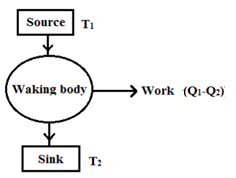(Surroundings)

Generally, the surrounding atmosphere acts as a sink for a heat engine.

Work done: W = Q1 – Q2

Thermal efficiency:

(i) $\eta =\frac{W}{{{Q}_{1}}}$

(ii) $\eta =\frac{{{Q}_{1}}-{{Q}_{2}}}{{{Q}_{1}}}=1-\frac{{{Q}_{2}}}{{{Q}_{1}}}$

## Refrigerator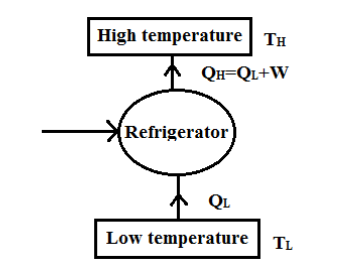A refrigerator is a cyclically operating energy-consuming device. Its main objective is to refrigerate a body with the help of ext. work. Heat is removed from the low-temperature body and is rejected to the high-temperature body. Generally, the surrounding atmosphere acts as the high-temperature body for a refrigerator.

Coefficient of Performance:

$\frac{{{Q}_{L}}}{W}=\frac{{{Q}_{L}}}{{{Q}_{H}}-{{Q}_{L}}}$

In the case of Carnot’s engine refrigerator,

$cop=\frac{{{T}_{L}}}{{{T}_{H}}-{{T}_{L}}}$

## Working of a Heat Pump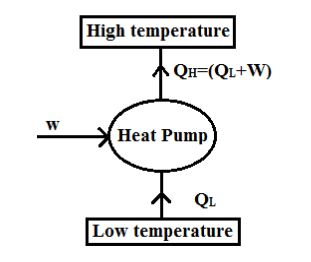A heat pump is a cyclically operating energy-consuming device. Its main objective is to heat a body with the help of ext work, heat is removed from the low temp body and is added to the high-temperature body. Generally, the surrounding atmosphere acts as a high-temperature body for the heat pump.

When we use a heat pump in heating mode, it follows the basic refrigeration-type cycle used by a refrigerator or an air conditioner. The only condition is that it occurs in the opposite direction. Heat is released into the conditioned space rather than the surrounding environment. In such a case heat pumps generally draw heat from the cooler external air or the ground.

$cop=\frac{{{Q}_{H}}}{W}=\frac{{{Q}_{H}}}{{{Q}_{H}}-{{Q}_{L}}},for\,a\,carnot\,heat\,pump,\,cop=\frac{{{T}_{H}}}{{{T}_{H}}-{{T}_{L}}}$

Note: When both the heat pump and the refrigerator are running between the same temperatures, the cop of a heat pump is always > cop off refrigeration.

$co{{p}_{HP}}=1+co{{p}_{R}}$

It the heat engine and the refrigerator are running between the same temperatures then the relation between the thermal efficiency η and cop is given by

$\eta =\frac{1}{1+co{{p}_{R}}}$

During the heating mode, the evaporator is the outdoor coil while the condenser is the indoor one. The refrigerant carries the thermal energy from the outside to the indoors. The vapour temperature is compressed within the pump. The indoor coil then moves the thermal energy to the indoor air.

In cooling mode the cycle is almost the same except in this case, the outdoor coil is used as the condenser and the indoor coil acts as the evaporator.

## Law of Thermodynamics

There are several statements given for 2nd law of thermodynamics, but the essence of all these statements is:

(i) No spontaneous process will take place in rev direction on its own.

### Kelvin-Planck Statement of 2nd Law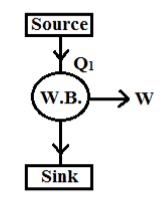It is impossible for any cyclically operating device (Heat engine), to convert all the heat received from a single thermal reservoir into work. That means out of the heat supplied only some amount is converted into work and the remaining heat should be rejected to 2nd thermal reservoir.

Therefore, according to this statement, the presence of a 2nd thermal reservoir is necessary for the running of a heat engine. As the work-output is always < energy input (i.e. W < Q1).

The thermal efficiency $(\eta )$ of the heat engine cannot be equal to unit $(i.e.\,\eta <1)$.

### Clausius Statement of 2nd Law

It is impossible (refrigerator (or) Heat pump) for any cyclically operating device to transfer heat from a low-temperature body to a high-temperature body without the help of an external agency.

That means, if you want to transfer heat from a low-temperature body to a high-temperature body, it is possible, only with the help of ext work. As always, external work is required as input. The cop of the refrigerator (or) heat pump cannot be equal to ∞.

Transfer of heat from a hot body to a cold body is a spontaneous process. According to Clausius’ statement, this spontaneous process will not take place in the rev directions on its own.

#### Violation of Clausius Statement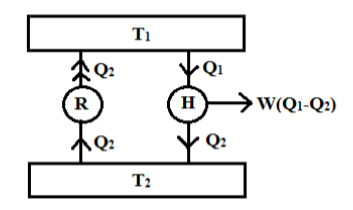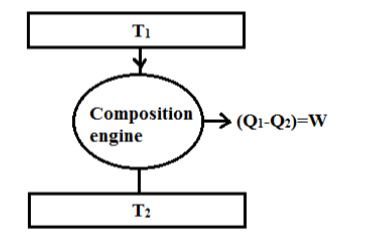The refrigerator is removing an amount of heat ‘Q2’ from the cold reservoir at temperature ‘T2’ and is rejecting heat to the hot reservoir on its own. Thus it violates the Clausius statement.

Meanwhile, a heat engine running between the same reservoirs is receiving an amount of heat Q1 from the hot reservoir and rejecting an amount of Q2 to the cold reservoir. This heat engine is not violating any statement of 2nd law.

If both the devices are having the same ‘T’ per cycle then the combination of them i.e. the composite engine is clearly violating the Kelvin Planck’s statement of 2nd law. When the heat engine is converting all the heat ‘Q1’ into work ‘W’ it also violates the statement.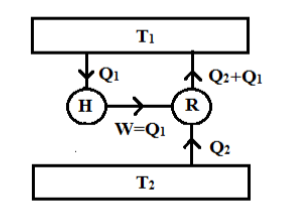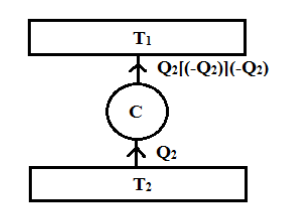Alternatively, the work output of the heat engine is driving a Carnot refrigerator as shown in the figure. The Carnot refrigerator is removing an amount of heat ‘Q2’ from the cold at temp ‘T2’ and rejecting the hot reservoir, ‘T1’ thus not violating and statement of 2nd law.

Both the devices have the same time period per cycle the combination of the engines i.e. composite engine violating the Clausius statement of 2nd law. When the Kelvin – Planck statement is violated, as a result, the Clausius statement is also violated and vice-versa. Both statements are considered to be equivalent.